Jul 27, 2016

Law of Large Numbers Failed

Problem:
There are two maternity hospitals in a town with 50 and 500 beds. Given full occupancy on a particular day, which of these hospitals is more likely to have equal no of boys and girls given probability of boys = probability of girls ?

What would the answer intuitively be by #‎LawOfLargeNumbers‬? You would see #LawOfLargeNumbers does not seem to work here. How should the statement be positioned for #LawOfLargeNumbers to work?

1.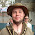I don't really see the connection to the LLN when you think the problem per se. It should be bleeding obvious that the smaller hospital is more likely to have equal numbers born. Even without calculating the binomial probabilities one can understand that greater number of possible outcomes makes any single one like 250-250 less likely. But admittedly, the fractional numbers fluctuate less with greater sample size.

2.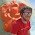The smaller hospital is more likely have an equal number of each sex. The respective probabilities are (50 choose 25) * (1/2)^50 ≈ 11% and (500 choose 250) * (1/2)^500 ≈ 3.6%.

The law of large numbers implies that as more and more babies are born, each with an independent 50/50 chance of being male of female, the proportion of males will converge to 50%. However, the law says nothing about this proportion equalling 50% exactly. The difference between converging to and equalling is a subtle one. To illustrate the difference, as x gets bigger and bigger, the number 1/x gets closer and closer to zero, but never actually equals zero. This is what happens with the Law of Large Numbers: the proportion of boys will get closer and closer to 50% over time, but not necessarily equal it.

To make the "intuitive" conclusion correct, you would have to ask: "Which hospital has a higher probability of having an approximately equal number of boys and girls?", where approximately equal means, say, that the proportion of boys is between 48% and 52%. However, this fact is better proved with the Central Limit Theorem, which specifically says that says not only that the proportion converges towards 50%, but that the error is approximately normally distributed and that the variance decreases (so being close to the mean is more likely).

3.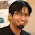The law of large numbers tells us that the expected number of boys/girls is concentrated in a width of O(sqrt(n)) around the mean. Heuristically, assuming it's a constant in this region, we deduce that the probability of getting exactly the same numbers is roughly 1/\sqrt(n), and hence larger for the 50 bed hospital.

The same answer can be derived by using a sterling approximation for the probability.

Here is an alternate view, you can think of taking the same distribution (gaussian without the tails), and splitting it into n buckets (n = 50, 500). The probability that we get equal numbers is just the central bucket, which since it gets smaller as n gets large, the probability must go down with n.

4.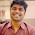Pr_50 = 50!/(25!*25!)/2^50
Pr_500 = 500!/(250!*250!)/2^500

Pr_50 > Pr_500.

If Nb is the number of boys and Ng is the number of girls,
Law of Large Numbers only claims that Pr( (Nb - Ng)/(Nb + Ng) ) approaches 0.

It does not claim about Pr( Nb = Ng ). It can happen that with increasing 'n', the difference increases but the ratio of differences to 'n' almost surely approaches 0.

5.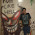I can't see why law of large numbers won't work here . The hospital with 50 beds is expected to have more variation from the expectation than the hospital with 500 beds.

6.One should ask which hospital will have proportion of boys closer to proportion of girls. LLN says sample mean to approach expected value which here means fraction of boys or girls will be closer to half in case of 500 beds, although the difference b/w boys and girls itself may rise.

7.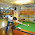I think this can be computed directly. Suppose there are 2n beds. Then the distribution of boys is a binomial with success probability p=0.5. Let the random variable X= number of boys in the hospital. Then number of girls is automatically 2n-X. So,
P(X=n)=\binomial(2n,n)(0.5)^(2n)
For n=25 : 0.1122751726592170485
For n=250 : 0.035664646
So we see that it is greater in case of n=25!!!
Lets look at the LLN for this problem. The LLN tells us only about the average behavior that is asymptotically on average every bed will be occupied by 0.5 boys. That is if X_i=1 if bed i has a boy and 0 o/w, then almost surely \sum_{i=1}^{2n}X_i converges to 0.5 . But LLN does not tell anything about the exact probability of the event. So we should be careful about how to apply the law of large numbers!!

8.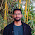How does Law of Large numbers relate to the question posed? Though there is a increase in the number of beds, the probability we calculate with Bernoulli distribution is a logical deduction rather than a inductive assumption. Law of large numbers expresses the guarantee of the axiomatic probability calculated to match with the classic frequency representation of probability. So, LLN doesn't apply here at all for it to fail. Am I missing something?

Fraction Brainteaser

Source: Sent to me by Gaurav Sinha Problem: Siddhant writes a Maths test and correctly answers 5 out of 6 Arithmetic questions and 20...